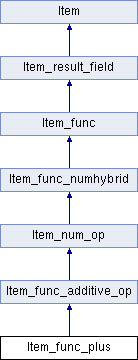My Project
Item_func_plus Class Reference
Inheritance diagram for Item_func_plus:List of all members.

## Public Member Functions

Item_func_plus (Item *a, Item *b)
const char * func_name () const
longlong int_op ()
Performs the operation that this functions implements when the result type is INT.
double real_op ()
Performs the operation that this functions implements when the result type is REAL.
my_decimaldecimal_op (my_decimal *)

## Member Function Documentation

 my_decimal * Item_func_plus::decimal_op ( my_decimal * decimal_value ) ` [virtual]`

Calculate plus of two decimals.

Parameters:
 decimal_value Buffer that can be used to store result
Return values:
 0 Value was NULL; In this case null_value is set \:: Value of operation as a decimal

Implements Item_func_numhybrid.

 longlong Item_func_plus::int_op ( ) ` [virtual]`

Performs the operation that this functions implements when the result type is INT.

Returns:
The result of the operation.

Implements Item_func_numhybrid.

 double Item_func_plus::real_op ( ) ` [virtual]`

Performs the operation that this functions implements when the result type is REAL.

Returns:
The result of the operation.

Implements Item_func_numhybrid.

The documentation for this class was generated from the following files: